## A chemist uses hot hydrogen gas to convert chromium(III) oxide to pure chromium. How many grams of hydrogen are needed to <

Question

A chemist uses hot hydrogen gas to convert chromium(III) oxide to

pure chromium. How many grams of hydrogen are needed to

convert 76 grams of chromium(III) oxide, Cr203?

in progress 0
5 months 2021-08-15T15:54:00+00:00 1 Answers 33 views 0

## Answers ( )

1. Answer: 3.024 g grams of hydrogen are needed to  convert 76 grams of chromium(III) oxide,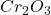Explanation:

The reaction equation for given reaction is as follows.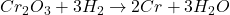Here, 1 mole ofreacts with 3 moles of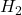.

As mass of chromium (III) oxide is given as 76 g and molar mass of chromium (III) oxide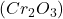is 152 g/mol.

Number of moles is the mass of substance divided by its molar mass. So, moles ofis calculated as follows.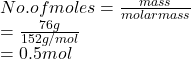Now, moles of.given by 0.5 mol ofis calculated as follows.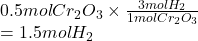As molar mass ofis 2.016 g/mol. Therefore, mass ofis calculated as follows.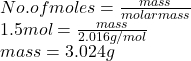Thus, we can conclude that 3.024 g grams of hydrogen are needed to  convert 76 grams of chromium(III) oxide,.